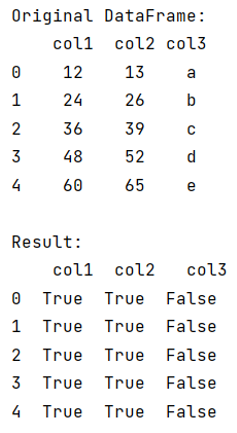# Finding non-numeric rows in dataframe in pandas

Given a Pandas DataFrame, we have to find non-numeric rows.
Submitted by Pranit Sharma, on July 23, 2022

Pandas is a special tool that allows us to perform complex manipulations of data effectively and efficiently. Inside pandas, we mostly deal with a dataset in the form of DataFrame. DataFrames are 2-dimensional data structures in pandas. DataFrames consist of rows, columns, and data.

## Problem statement

Given a Pandas DataFrame, you have to find the non-numeric rows from it.

Sometimes, while dealing with a large data set, we deal with every kind of data type but we need some specific data types. For instance, we sometimes need to find non-numeric rows in DataFrame.

## Find non-numeric rows in dataframe in pandas

To find non-numeric rows in dataframe in pandas, we will first use the map() method which will help us to traverse each value of DataFrame so that we can check the value at the same time. We will check the value using np.isreal() method. This method returns Boolean value. If it returns True, it means that the value is numeric and if False is the result, then the value is non-numeric. In this way, we can check that a row is numeric or non-numeric values in it.

Let us understand with the help of an example,

## Python program to find non-numeric rows in dataframe in pandas

```# Importing pandas package
import pandas as pd

# Importing numpy package
import numpy as np

# Creating a Dictionary
d = {
'col1':[12,24,36,48,60],
'col2':[13,26,39,52,65],
'col3':['a','b','c','d','e']
}

# Creating a DataFrame
df = pd.DataFrame(d)

# Display original DataFrame
print("Original DataFrame:\n",df,"\n")

# Checking values
result = df.applymap(np.isreal)

# Display result
print("Result:\n",result)
```

## Output

The output of the above program will be: